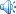# 北师大版数学二年级下册第五单元试卷• 发布时间：2012-04-05 05:29:00
• 发布者：吾爱
• 微信号：wuaiyazhu.
• 浏览量：
• Tags：
•公告：

1、在括号里填上合适的单位名称。

2 、 1cm=( )mm 1m=( )dm 1km=( )m
4km=( )m 2000m=( )km 9dm=( )cm
500cm=( )m 30mm=( )cm 7cm=( )mm
3、在○里填上＞、＜或＝。
14cm○20mm 100mm○10cm 8毫米○7厘米
1m○8dm 2千米○2100米 1厘米○10毫米
4、我会计算。
15米+9米=（ ） 46厘米—26厘米=（ ）
48千米+32千米=（ ） 1米—20厘米=（ ）
3千米—2千米=（ ） 5分米—50厘米=（ ）

1、一张床长约22dm。 ( )
2、明明的身高150cm，红红的身高15dm，两人一样高 。 ( )
3、测量一段路的长度，可以用km, m做单位。 ( )
4、飞机每小时飞行800米。 ( )

1口算。（16分）
9×9= 85—35= 7×8= 3×5+7=
45÷9= 45+20= 100—60= 21÷3+4=
8×5= 26+34= 36÷9= 8×6—9=
63÷7= 66—45= 80+50= 81÷9+1=
2用竖式计算。（12分）
90—38= 78+34= 25÷5=

50÷7= 63÷9= 70÷8=

1、画一条长65mm的线段
2、画一条比5cm长25mm的线段
3、量一量 .
( )
cm

1、一根钢管长50米，如果要剧成8米一断，需要锯几次？（7分）

2、小军的身高是1米45厘米，小强的身高是1米65厘米。

3、一块花布长60分米，做一套衣服用9分米，至多能做几套衣服？（7分）

4、这跟绳子长70米，每8米剪成一段，可以剪成几段？（6分）